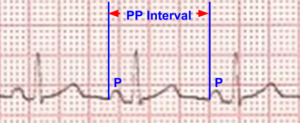# PP interval

## PP intervalPP interval on ECG

PP interval is the interval between the P waves due to atrial depolarization (measured from the onset of one P wave to the onset of the next P wave). We can calculate the atrial rate from the PP interval. In sinus rhythm, PP and RR intervals are the same. Hence atrial rates and ventricular rates are not calculated separately. But when there is AV (atrioventricular) dissociation as in complete heart block, atrial rate is different from the ventricular rate. In complete heart block, PP interval is shorter than the RR interval. This means that atrial rate is higher than the ventricular rate. In ventricular tachycardia with interference AV dissociation, PP interval is longer than the RR interval, indicating that atrial rate is lower than ventricular rate. Both these intervals are nearly identical in isorhythmic AV dissociation.

P-P interval is measured in milliseconds. Atrial rate is calculated by dividing 60000 by the measured P-P interval. 60000 milliseconds corresponds to one minute, hence atrial rate obtained is in beats per minute. Alternatively PP interval can be measured in millimeters. With a standard ECG with paper speed of 25 millimeters per second, atrial rate is obtained by dividing 1500 by the PP interval in millimeters. This approach is more commonly adopted while calculating the atrial rate from the ECG as it avoids conversion of intervals initially into milliseconds. In some of the modern devices, there is provision for automatic calculation of heart rates, but it seldom calculates the atrial rate separately.

PP interval can vary slightly in normal individuals from cycle to cycle due to respiratory sinus arrhythmia. The atrial rate increases in inspiration and decreases in expiration. This is because of cyclical changes in left ventricular output with respiration and reflex changes in heart rate. In normal adults, respiratory sinus arrhythmia can cause a variation in P-P interval up to 3 mm (120 ms).

Respiratory sinus arrhythmia is quite common in normal children and is of no clinical significance.  Exaggerated respiratory sinus arrhythmia is a common cause for irregular pulse in children. Another rare form of non respiratory sinus arrhythmia which occurs in complete heart block is known as ventriculophasic sinus arrhythmia [1,2].

## Reference

1. Wilson FN, Robinson AC. Two cases of complete heart block showing unusual features Arch Int Med. 1918; 21:166.
2. Dadu RT, McPherson CA. The ventriculophasic response revisited: analysis of clinical correlations using a new proposed definition derived in pacemaker patients. Clin Cardiol. 2012 Jan;35(1):21-5.